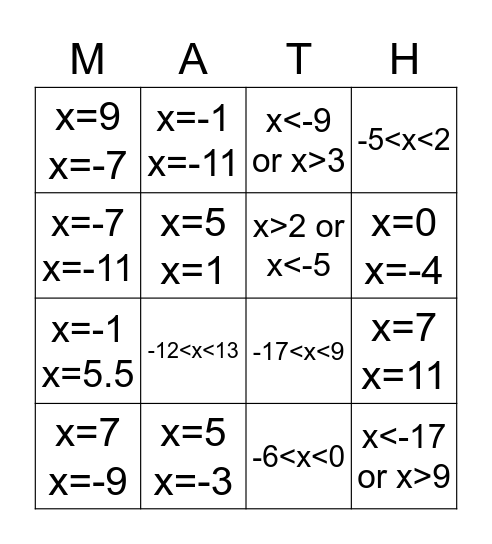Algebra 1 4.6 ReviewThis bingo card has 24 words: x=6 x=-6, x=7 x=-7, x=0 x=-4, x=0 x=-18, x=-7 x=-11, x=9 x=-7, x=5 x=1, x=-1 x=-11, x=5 x=-3, x=23 x=-3, x=-1 x=5.5, -17<x<9, x>10 or x<0, -9<x<7, x>2 or x<-5, x>5 or x<-9, -6<x<0, x<-9 or x>3, -12<x<13, x=7 x=11, x=7 x=-9, x<-17 or x>9, -5<x<2 and x<-6 or x>0.

Play Online